# Scipy image processing and manipulation through Python

In this tutorial, you are going to learn about the image processing and manipulation through SciPy module. To make the image manipulation and processing, firstly we have to import all the modules.

```from scipy import misc,ndimage
from matplotlib import pyplot as plt
import numpy as np```

The basic operations in image processing and manipulations are: displaying the image, grayscale the image, blur the image, crop the image, rotate the image and etc.

• Display Image
• Grayscale Image
• Rotate an Image
• Crop an Image
• Blur Image

## Image display in Python using SciPy and matplotlib

To display the image, some inbuilt images are saved in the misc sub-package. You can access these images and plot them on the scale with the help of the methods of matplotlib module.

```from scipy import misc
from matplotlib import pyplot as plt
import numpy as np
f1=misc.face()  # to get the image
plt.imshow(f1)
plt.show()      # to show the image
```

Output:-```from scipy import misc
from matplotlib import pyplot as plt
import numpy as np
f2=misc.ascent()
plt.imshow(f2)
plt.show()
```

Output:-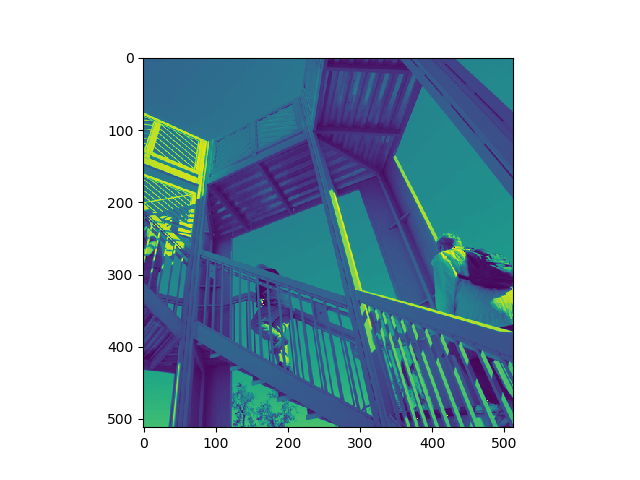## Grayscale image in Python using SciPy and matplotlib

The color of the image can be the change with the help of gray parameter of the face.
The graphical axis can be removed with the plt.axis(‘off’).

```from scipy import misc
from matplotlib import pyplot as plt
import numpy as np
f1=misc.face(gray=True)
plt.imshow(f1)
plt.axis('off')
plt.show()
```

Output:-## Image rotation in Python using SciPy and matplotlib

Image rotation is done with the ndimage sub-module of SciPy. The rotate() method will rotate the image to the mentioned angle in the parameter.

```from scipy import misc,ndimage
from matplotlib import pyplot as plt
import numpy as np
f1=misc.face()
ro=ndimage.rotate(f1,45)
plt.imshow(ro)
plt.show()
```

Output:-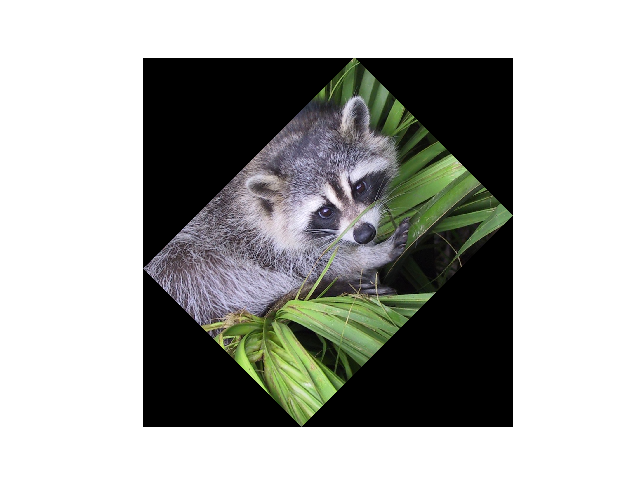The fluipud() method will flip the image from up to down and down to up with respect to the image position.

```from scipy import misc,ndimage
from matplotlib import pyplot as plt
import numpy as np
f1=misc.face()
flip = np.flipud(f1)
plt.imshow(flip)
plt.show()
```

Output:-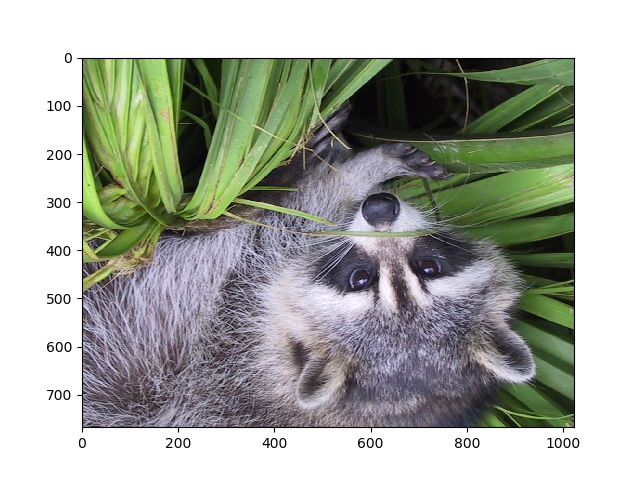## Cropping the image in Python using SciPy and matplotlib

The size of the image can be altered. The shape will get the size of the image after that you can crop it by using slicing.

```from scipy import misc,ndimage
from matplotlib import pyplot as plt
import numpy as np
f1=misc.face()
lx,ly,lz=f1.shape
crop = f1[lx // 4: - lx // 4, ly // 4: - ly // 4]
plt.imshow(crop)
plt.show()
```

Output:-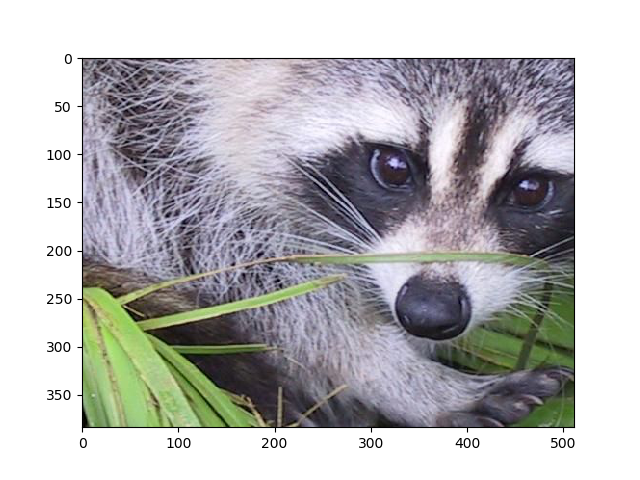## Blur image in Python using SciPy and matplotlib

The gaussian_filter()  will blur the image. The value of sigma will increase the blur effect in the image.

```from scipy import misc,ndimage
from matplotlib import pyplot as plt
import numpy as np
f1=misc.face()
blur=ndimage.gaussian_filter(f1,sigma=4)
plt.imshow(blur)
plt.show()
```

Output:-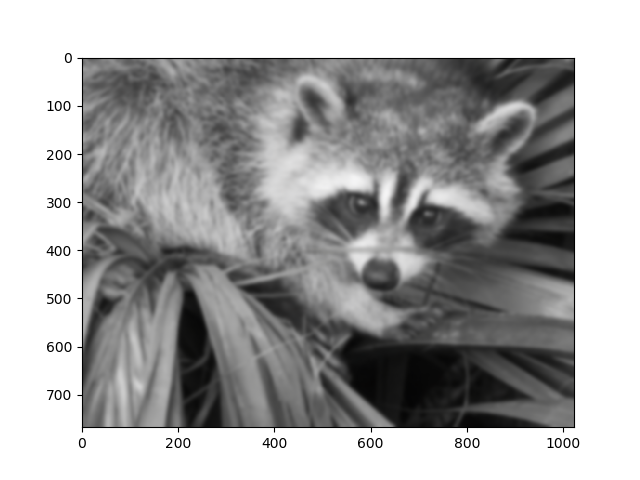Check out the other tutorials on Python:

Seaborn module of Python

Shallow copy and Deep copy in Python

### One response to “Scipy image processing and manipulation through Python”

1.lee smith says:

Thanks.
Can you provide further URLs using ndimage, scipy, and matplotlib which manipulate images